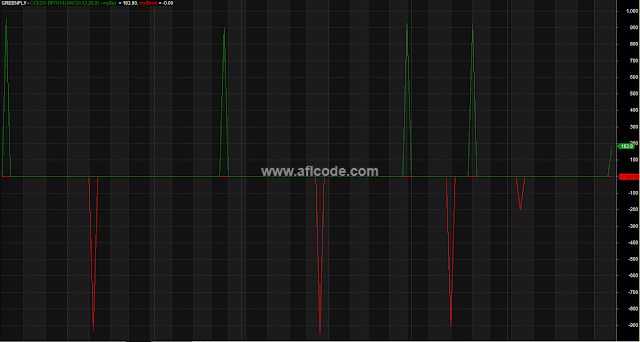### CCI MACD DPO Combined SystemCCI MACD DPO Combined System

```//www.aflcode.com
_SECTION_BEGIN("CCI + DPO + MACD");
// Written by Barry Scarborough 2/15/05
// Updated 8/10/05 - corrected DPO formula
//
// This Signal was discussed on the VV forum. I am not sure who developed it.
// It combines the three indicators, MACD, DPO AND Trix into one timing Signal.
// A Signal will only occur when all three conditions exist.
// The two timing signals are buy/cover and short/sell signal. The purpose is to keep the user in the market all the time.

// macd
// Param allows changing parameters without changing the code, the default are the standard defaults for MACD
r1 = Param( "MACD Fast avg", 12, 2, 200, 1 );
r2 = Param( "MACD Slow avg", 26, 2, 200, 1 );
r3 = Param( "MACD Signal avg", 9, 2, 200, 1 );
upMacd = IIf(MACD(r1,r2) > Signal(r1,r2,r3), 1, 0); // up and down signal

// dpo - detrended price oscillator
n = Param("DPO period", 14, 2, 100, 1);
p = int( ( n / 2) + 1);
movA = MA(C, n);
dpo = C -  Ref(movA, -p);
upDpo = dpo > 0;
dnDpo = dpo < 0;

// CCI
periods = Param("CCI period", 20, 2, 100, 1 ); // default is 10
myCCI = CCI( periods );

// signal conditions
myBuy = upDpo AND upMacd AND myCCI > 0;
myShort = dnDpo AND !upMacd AND myCCI < 0;

Short = Sell = ExRem(myShort, myBuy);

Plot( Buy * C, "CCI(" + NumToStr(periods,1.0) +
") DPO(" + NumToStr(n,1.0) +
") MACD(" + NumToStr(r1,1.0) + "," + NumToStr(r2,1.0) + "," + NumToStr(r3,1.0) +